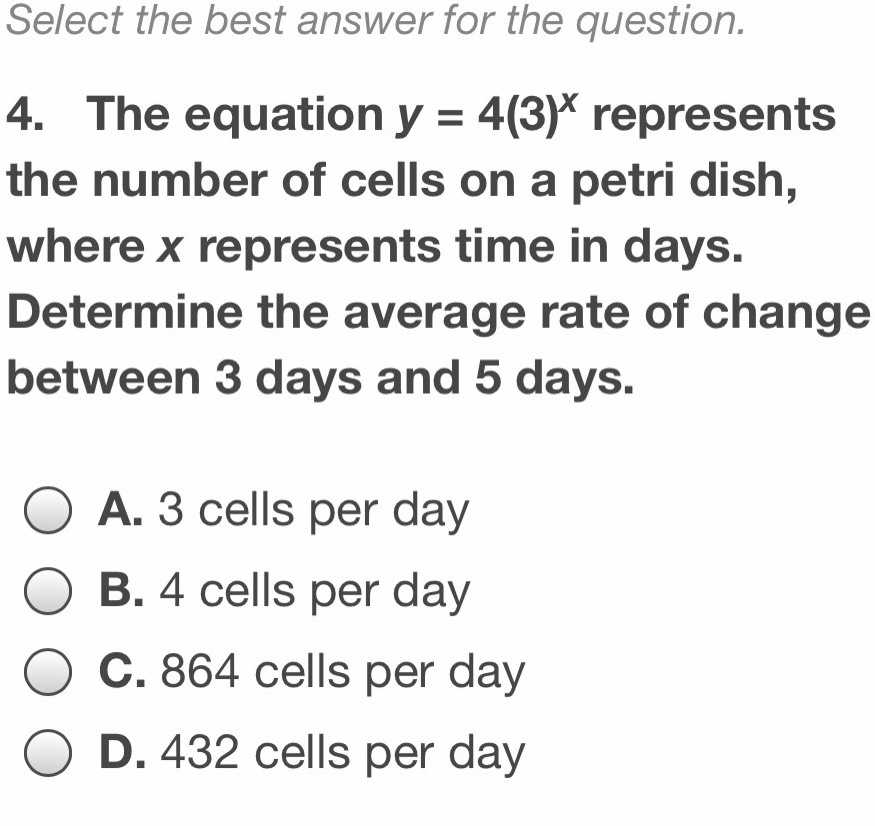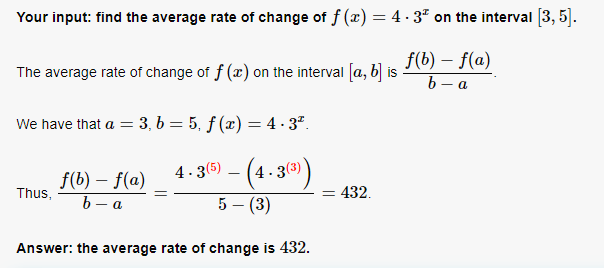### ¿Todavía tienes preguntas de matemáticas?

Pregunte a nuestros tutores expertos
Algebra
Pregunta4. The equation $$y = 4 ( 3 ) ^ { x }$$ represents the number of cells on a petri dish, where $$x$$ represents time in days. Determine the average rate of change between $$3$$ days and $$5$$ days.

A. $$3$$ cells per day

B. $$4$$ cells per day

C. $$864$$ cells per day

D. $$432$$ cells per day